SherpaGUEST POST

# Maths Skills to Pass the A-level Biology ExamMaths skills made up 10% of the marks for A-Level Biology, so it is worth brushing up on some of the key concepts to make sure you’re not missing out on all important marks in your exams.

The main reason students struggle with the maths aspect of A-Level Biology is that they don’t understand what is expected of them. The maths content is a lot less daunting if you give 5 minutes to appreciate it, so let’s have a look at what's needed...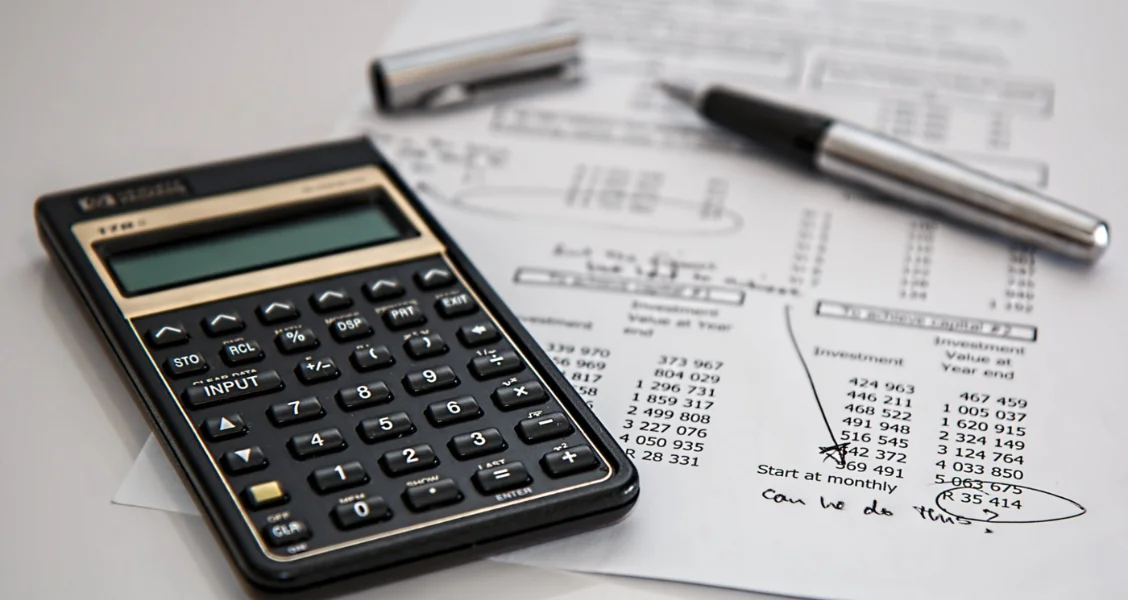### Arithmetic & Numerical Computation

• Using standard form to represent the size of measurements e.g. size of organelles.
• Converting between units to represent the size of cells or organelles.
• Using ratios, fractions and percentages.
• Calculating the number of bacteria grown over a period of time using powers, exponentials and logs.

### Handling Data

• Reporting data to significant figures.
• Finding means such as the mean number of stomata on a leaf as well as medians and modes.
• Understand measures of dispersion and standard deviation.
• Calculate percentage error in data.

### Statistics

• Carry out the 3 main statistical tests – Chi-Squared test, Student’s t-test and Correlation Coefficient.
• Understand how to use the terms probability and chance.

### Equations

• Recall the Magnification Equation and be able to rearrange the formula.
• Recall equations for Pulmonary Ventilation Rates, Cardiac Output, Net Primary Production (NPP) and Net Production of Consumers (N), Hardy Weinberg equations.
• Use equations when they are given in the exam e.g. Simpson’s Index of Biodiversity.

### Graphs

• Plot two variables on a graph from experimental data.
• Determine the intercept of a graph where it crosses the Y axis e.g. compensation points in plants.
• Interpret scattergrams to identify correlations e.g. the effect of lifestyle on health
• Interpreting data from graphs e.g. breathing rate, heart rate from ECGs, rate of activity etc.
• The concept that y = mx + c is a linear relationship (straight line). You may be asked to sketch graphs with linear relationships.
• Calculate rates from graphs showing a linear change and use a tangent to calculate the rate of change.

### Geometry

• Calculate the circumference and area of a circle e.g. to work out zones of inhibition on agar plates.
• calculate the surface area and volume of rectangular prisms, cylindrical prisms and spheres e.g. to find the surface area to volume ratios (SA:V) of certain organisms or cells.

While this might seem like a lot, many aspects you have already faced simply by learning the content of the course. Let us break down the first of these topics to highlight some of the key maths skills you will need to have mastered before you’re A-Level Biology exam.## Topic 1 - Arithmetic & Numerical Computation

### Converting between Units & Standard Form

Say we have used the magnification equation to work out that the actual size of an animal cell is 0.0083mm. When we talk about cells, we usually would use micrometres to express size, so you could convert millimetres to micrometres.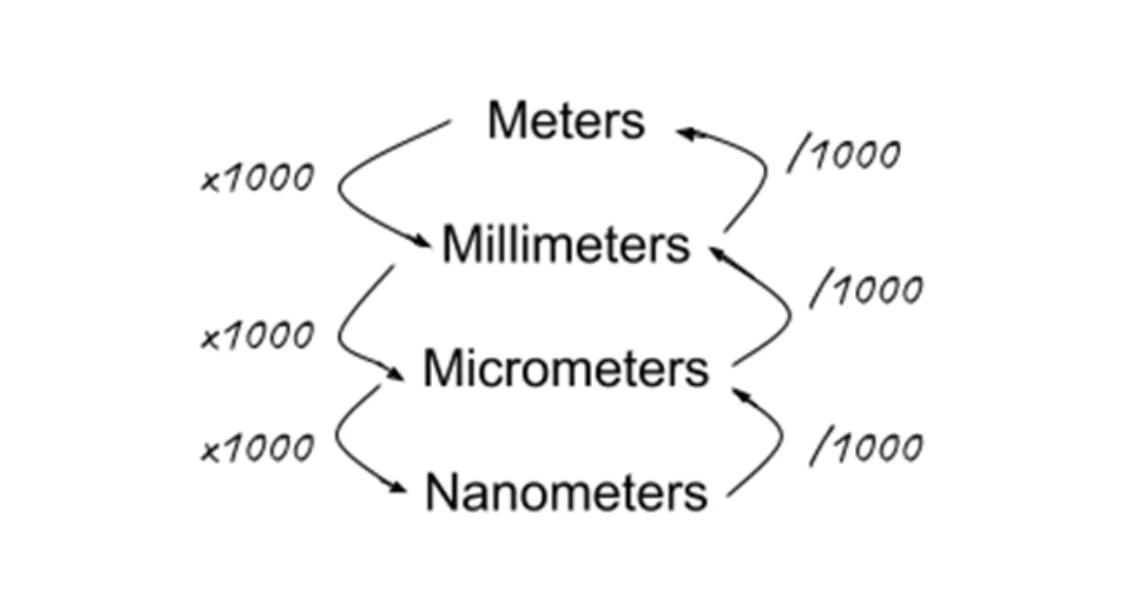Our answer would therefore be 8.3 micrometres – a much more sensible-looking number.

Alternatively, you may be asked to represent 0.0083mm in Standard Form.

Standard Form is a way to represent really big or really small numbers so that they don’t look at our place. All you have to do is move the decimal place until you create a number between 1 and 10.

For example, for 0.0083 we move the decimal place 3 spaces to the left to make a number between 1 and 10 (8.3) and as we moved them to the right, we write 8.3X10-3.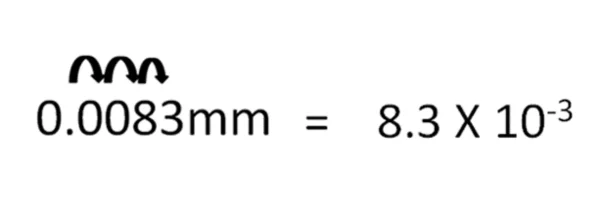### Now, can you do these yourself?

Task: Convert 0.0000365 into standard form

### Using Fractions, Ratios & Percentages

While these operations may be quite straightforward, it is worth pointing out scenarios where you might have to use them.

Fractions are seldom used in Biology, but it is worth remembering basics such as converting between these forms, for example, 25% = 0.25 = ¼

Ratios are a much more common way of representing data e.g. phenotypic ratios for genetic crosses and surface area to volume ratios (SA:V) for the topic of Exchange. Ratios can be found by dividing the first figure in a ratio by the second and putting it to 1.

Percentages are most commonly used when talking about two areas of maths:

1.  Percentage Change e.g. if a potato tuber takes up water via osmosis in an experiment, what is the change in mass of the potato tuber from the start of the experiment compared to the end? You will need to remember this equation for your exam:2.  Percentage Yield e.g. how much insulin could you produce from a population of genetically engineered bacteria compared to how much you actually made. Remember this equation for your A-Level exam: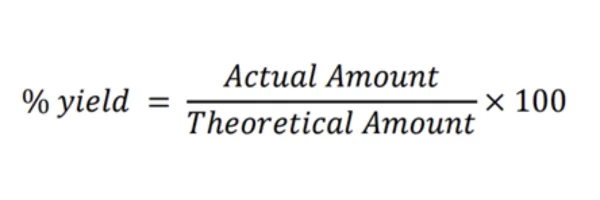### Now, can you do this yourself?

Task: In an experiment on osmosis, the weight of a beetroot cell before the experiment was 1.34g.

﻿

﻿After the experiment, it was 1.28g. Calculate the percentage change in mass of the beetroot cell.

### Calculating the Number of Bacteria using Powers, Exponentials and Logs

The main area this provides a challenge is when dealing with Bacteria divide exponentially by Binary Fission. The formula to calculate the number of bacterial cells present after n number of generations is: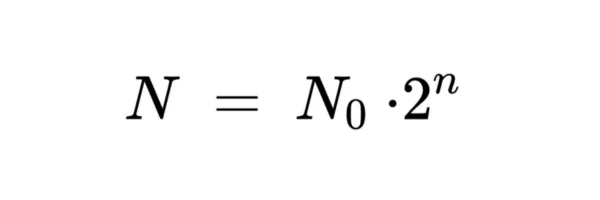For example, if we start with 1 bacterium and it divides 12 times, we can write 1 x 212 = 4096 bacterial cells present in the 12th generation.

Given some data, you may be asked to calculate how many bacteria are present after a certain number of divisions (generations).

### Now, can you do this yourself?

Task: A start population has 12 bacteria. The bacteria replicate once every 20 minutes. How many bacteria will be present after 2 hours 20 minutes?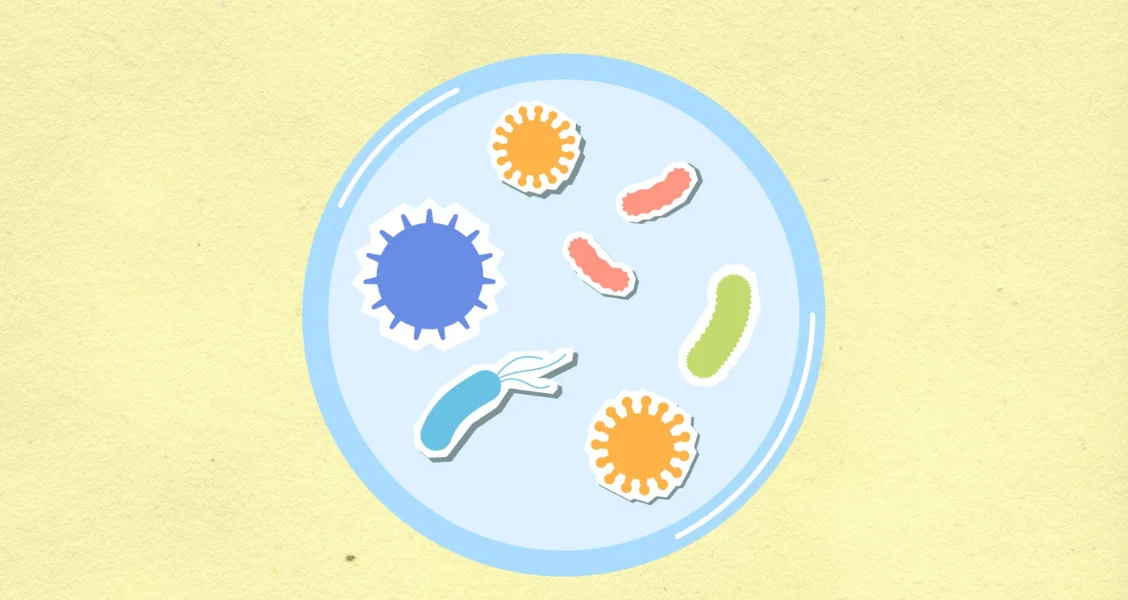Hopefully, that has given you a view of how the maths skills for A-Level Biology can be put into use. Below are some of the important maths aspects to be aware of in the other 5 main areas stated at the start.

## Topic 2 - Handling Data

### Significant Figures

If you are asked to give an answer to a certain number of significant figures, it simply means to round your number.

For example, giving 4.99 to 2 significant figures would be 5.0. There are two numbers (significant figures) in our answer. 0 is classed as a significant figure.

### Means, median and modes

• Mean = the sum of all the numbers divided by how many numbers there are
• Mode = the most common number
• Median = the middle value

### Measures of Dispersion and Standard Deviation

• Range = the difference between the highest and lowest number
• Standard Deviation = how close the spread of the data is to the mean
• 68% of the data should lie within 1SD of the mean, while 95% should lie within 2SD

### Percentage Error

You may be asked to calculate the percentage error of measurement. This is made up of:

• Absolute uncertainty e.g. a piece of equipment may be accurate up to a certain point, for example, a thermometer can only take temperature correctly within a range of 0.5oC. This means any measurement might be wrong by this much on either side
• Quantity measured e.g. your actual measurement

You can then use the following formula: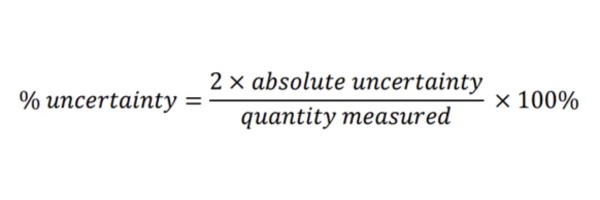## Topic 3 - Statistical Tests

Below is a summary of the stats tests you need to know for A-Level Biology. Often questions ask which would be the most appropriate test in a given situation. Use the data and information you are given to match them to the correct test.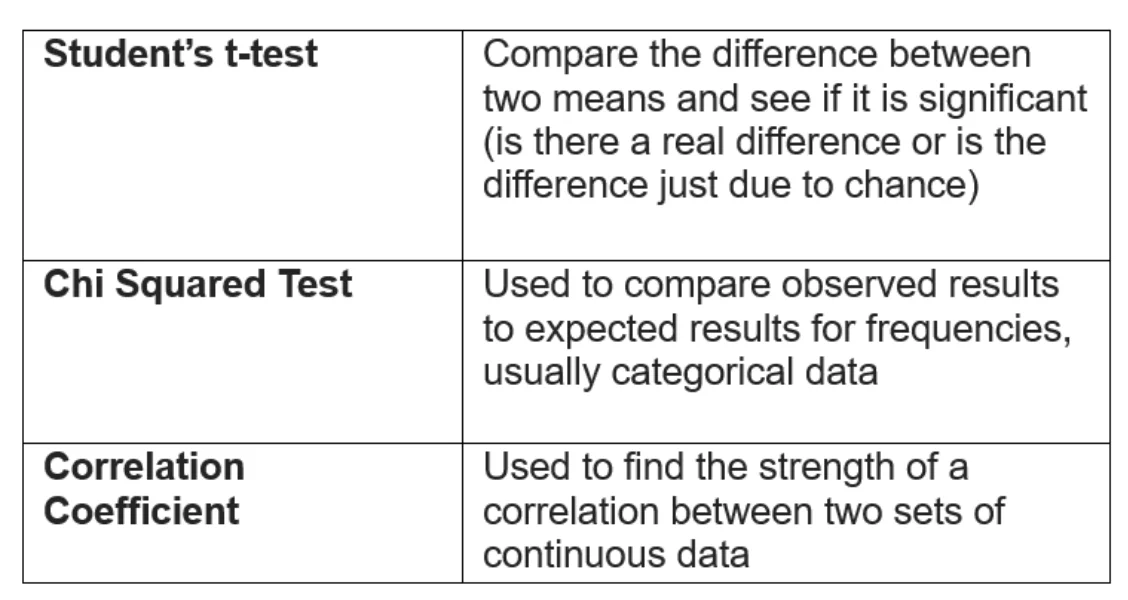### Understand the terms - Probability and Chance

Test values are compared at a 0.05 probability level. If your p-value is less than 0.05, it means there is less than a 5% probability that your results are due to chance and therefore you reject the null hypothesis and state there is some biological effect going on here.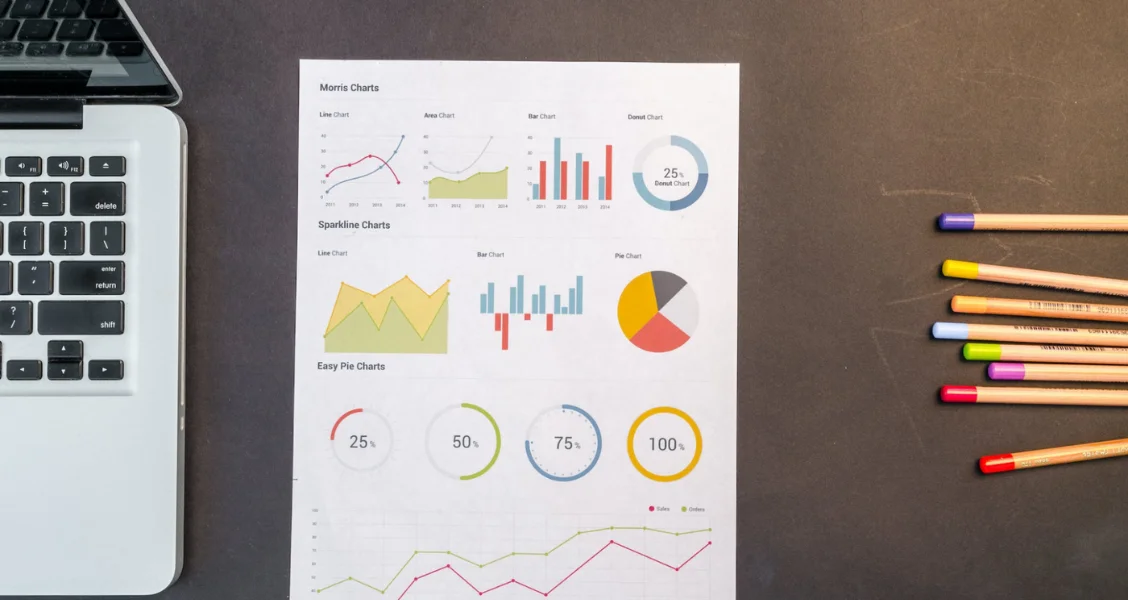## Topic 4 - Equations

Recall and be able to manipulate the following equations:

• Magnification = image size / actual size
• Pulmonary Ventilation Rate (PVR) = tidal volume x breathing rate
• Cardiac Output (CO) = stroke volume x heart rate
• Net Primary Production (NPP) = Gross Primary Production (GPP) – Respiration (R)
• Net Production of Consumers (N) = chemical energy stored in ingested food (I) – (chemical energy store lost to the environment in faeces [F] + respiratory losses [R]). In short – N = I - (F + R)
• Hardy Weinberg Equations for Gene Frequency and Allele Frequency (See Below).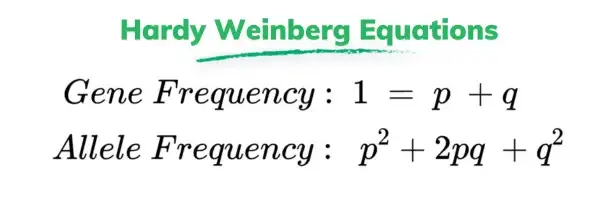The other equation you may be asked to use, but you do not have to remember, is that for Simpson’s index of biodiversity: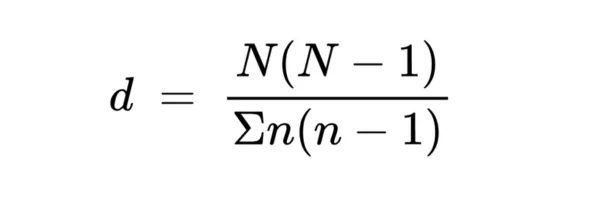## Topic 5 - Graphs

This maths section is more focused on practical aspects of data, rather than calculations.

You should feel comfortable interpreting different types of graphs:

• Bar Charts
• Histograms
• Scattergrams

Make sure you are happy that the X-axis runs along the bottom and the Y-axis runs up the side of a graph.

My advice is to use graphs that you see in your textbook and your past paper questions and spend a minute understanding what they are showing and that you understand how the information is being put across.## Topic 6 - Geometry

As annoying as it may seem, there are a few key formulas to remember here. Luckily, the only place they are likely to appear in your exam is when dealing with surface area to volume ratios!

Below are the most common formulae to be aware of:

• Calculate the circumference and area of a circle
• Calculate the surface area and volume of rectangular prisms, of cylindrical prisms and of spheres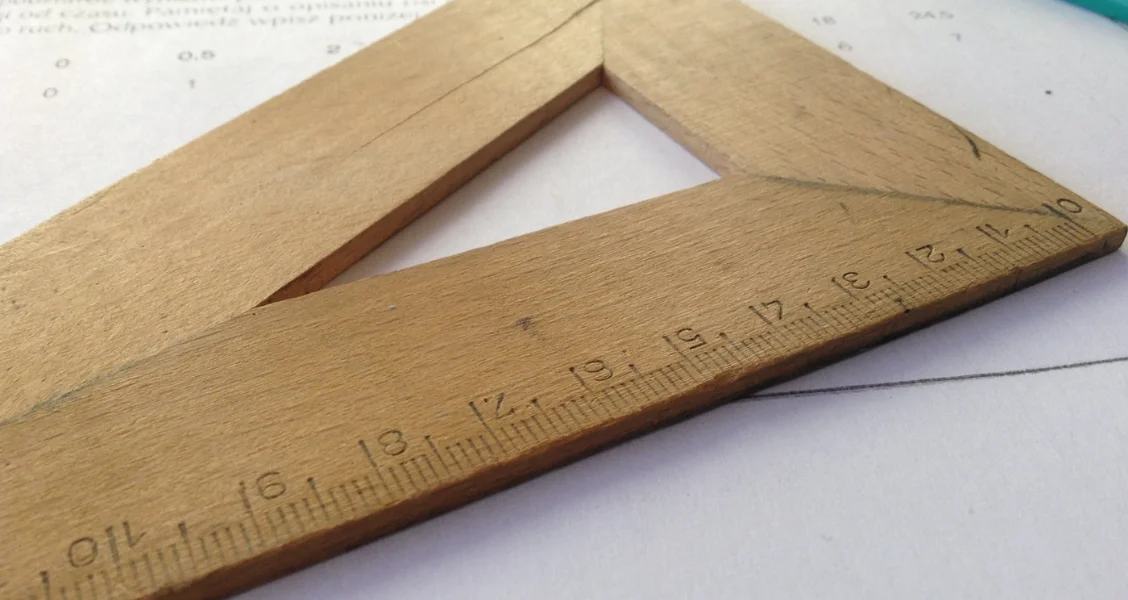## Example Questions

Let us look at a few exam questions and what the mark schemes can look like:

### Question 1

The replication rates of two bacteria were analysed. Bacterial strain A was found to replicate every 20 minutes whereas bacterial strain B replicates every 50 minutes.

After 1 hour 40 minutes what will the ratio of A to B be, given that both strains started dividing with the same number of bacteria? [2 marks]

• Strain A will replicate 5 times (100 minutes / 20 minutes) while strain B will replicate twice (100 minutes / 50 minutes) [1 mark]
• Ratio 5:2 [1 mark]

Out of the 250 bacteria in culture C, 15 of them were antibiotic-resistant. What percentage was not antibiotic resistant. [2 marks]

• 250 - 15 = 235 [1 mark]
• (235/250) x 100 = 94% [1 mark]

### Question 2

An image of an animal cell is 30 mm in size and it has been magnified by a factor of x4200. What is the actual size of the cell in micrometres? [3 marks]

• Write out the equation à A = I / M [1 mark]
• Put in the values à A = 30mm / 4200 so A = 0.0071mm [1 mark]
• Convert millimetres to micrometres à A = 0.0071 x 1000 so A = 7.1μm [1 mark]

##### Looking for a tutor?

Sherpa has hundreds of qualified and experienced UK tutors who are ready to help you achieve your goals. Search through our tutors and arrange a free 20 minute introduction through our industry-leading online classroom.

Find a Tutor